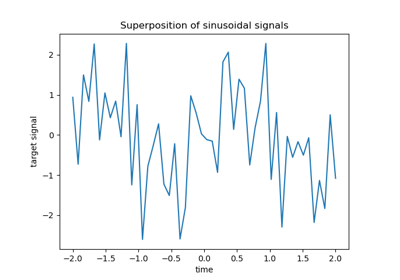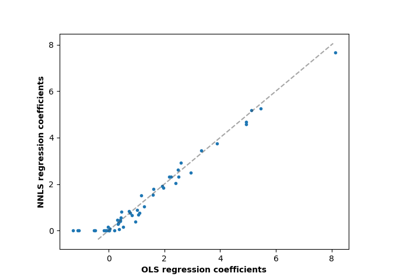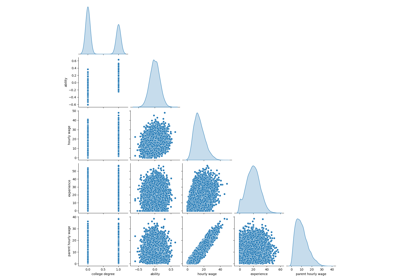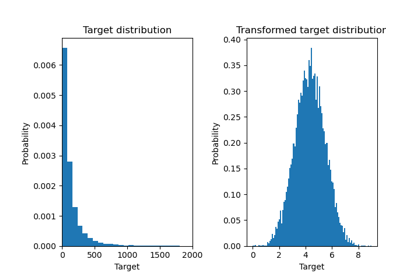# sklearn.metrics.r2_score¶

sklearn.metrics.r2_score(y_true, y_pred, *, sample_weight=None, multioutput='uniform_average', force_finite=True)[source]

$$R^2$$ (coefficient of determination) regression score function.

Best possible score is 1.0 and it can be negative (because the model can be arbitrarily worse). In the general case when the true y is non-constant, a constant model that always predicts the average y disregarding the input features would get a $$R^2$$ score of 0.0.

In the particular case when y_true is constant, the $$R^2$$ score is not finite: it is either NaN (perfect predictions) or -Inf (imperfect predictions). To prevent such non-finite numbers to pollute higher-level experiments such as a grid search cross-validation, by default these cases are replaced with 1.0 (perfect predictions) or 0.0 (imperfect predictions) respectively. You can set force_finite to False to prevent this fix from happening.

Note: when the prediction residuals have zero mean, the $$R^2$$ score is identical to the Explained Variance score.

Read more in the User Guide.

Parameters:
y_truearray-like of shape (n_samples,) or (n_samples, n_outputs)

Ground truth (correct) target values.

y_predarray-like of shape (n_samples,) or (n_samples, n_outputs)

Estimated target values.

sample_weightarray-like of shape (n_samples,), default=None

Sample weights.

multioutput{‘raw_values’, ‘uniform_average’, ‘variance_weighted’}, array-like of shape (n_outputs,) or None, default=’uniform_average’

Defines aggregating of multiple output scores. Array-like value defines weights used to average scores. Default is “uniform_average”.

‘raw_values’ :

Returns a full set of scores in case of multioutput input.

‘uniform_average’ :

Scores of all outputs are averaged with uniform weight.

‘variance_weighted’ :

Scores of all outputs are averaged, weighted by the variances of each individual output.

Changed in version 0.19: Default value of multioutput is ‘uniform_average’.

force_finitebool, default=True

Flag indicating if NaN and -Inf scores resulting from constant data should be replaced with real numbers (1.0 if prediction is perfect, 0.0 otherwise). Default is True, a convenient setting for hyperparameters’ search procedures (e.g. grid search cross-validation).

New in version 1.1.

Returns:
zfloat or ndarray of floats

The $$R^2$$ score or ndarray of scores if ‘multioutput’ is ‘raw_values’.

Notes

This is not a symmetric function.

Unlike most other scores, $$R^2$$ score may be negative (it need not actually be the square of a quantity R).

This metric is not well-defined for single samples and will return a NaN value if n_samples is less than two.

References

Examples

>>> from sklearn.metrics import r2_score
>>> y_true = [3, -0.5, 2, 7]
>>> y_pred = [2.5, 0.0, 2, 8]
>>> r2_score(y_true, y_pred)
0.948...
>>> y_true = [[0.5, 1], [-1, 1], [7, -6]]
>>> y_pred = [[0, 2], [-1, 2], [8, -5]]
>>> r2_score(y_true, y_pred,
...          multioutput='variance_weighted')
0.938...
>>> y_true = [1, 2, 3]
>>> y_pred = [1, 2, 3]
>>> r2_score(y_true, y_pred)
1.0
>>> y_true = [1, 2, 3]
>>> y_pred = [2, 2, 2]
>>> r2_score(y_true, y_pred)
0.0
>>> y_true = [1, 2, 3]
>>> y_pred = [3, 2, 1]
>>> r2_score(y_true, y_pred)
-3.0
>>> y_true = [-2, -2, -2]
>>> y_pred = [-2, -2, -2]
>>> r2_score(y_true, y_pred)
1.0
>>> r2_score(y_true, y_pred, force_finite=False)
nan
>>> y_true = [-2, -2, -2]
>>> y_pred = [-2, -2, -2 + 1e-8]
>>> r2_score(y_true, y_pred)
0.0
>>> r2_score(y_true, y_pred, force_finite=False)
-inf


## Examples using sklearn.metrics.r2_score¶Lasso and Elastic Net for Sparse Signals

Lasso and Elastic Net for Sparse SignalsLinear Regression Example

Linear Regression ExampleNon-negative least squares

Non-negative least squaresFailure of Machine Learning to infer causal effects

Failure of Machine Learning to infer causal effectsEffect of transforming the targets in regression model

Effect of transforming the targets in regression model